## Topic 6.3: Transformations to Linear Regression (Error Analysis)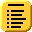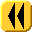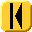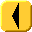Introduction Notes Theory HOWTO Examples Engineering Error Questions Matlab Maple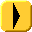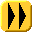To be done later, though one comment: when we convert exponential data to linear data, because we are taking the logarithm of each of the y values, the resulting curve minimizes the sum of the squares of the logarithms of the errors, not the sum of the squares of the errors themselves, so the fit will be much more tight for smaller values.